# Be sure to answer all parts. How many milliliters of a 0.24 M sucrose solution contain...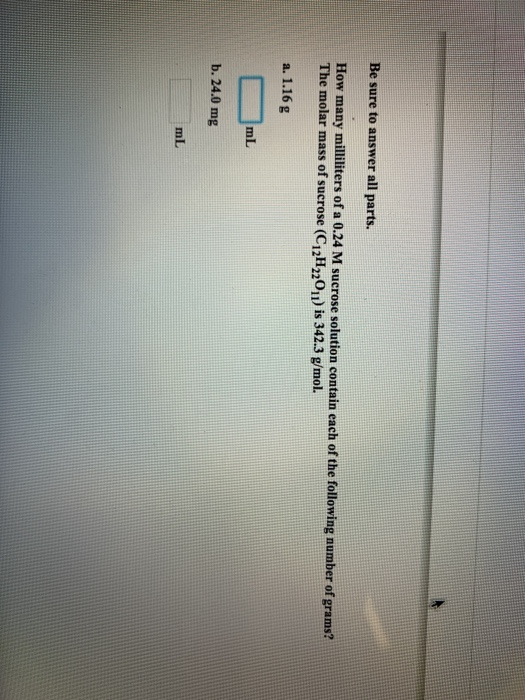Be sure to answer all parts. How many milliliters of a 0.24 M sucrose solution contain each of the following number of grams? The molar mass of sucrose (C12H22011) is 342.3 g/mol. a. 1.16 g ml b. 24.0 mg

Molarity of a solution is given as,

Molarity = moles/volume in liters

Number of moles = mass/molar mass

a. 1.16 g

Number of moles = 1.16/342.3

= 0.00339 moles

Volume = 0.24 M × 0.00339 mol

= 0.0008136 L

1 L = 1000 ml

Volume = 0.814 ml

b. 24 mg

1 mg = 0.001 g

24 mg = 0.024 g

Number of moles = 0.024/342.3

= 7.01 × 10^-5 mol

Volume = 7.01 × 10^-5 × 0.24

= 0.017 ml

#### Earn Coin

Coins can be redeemed for fabulous gifts.

Similar Homework Help Questions
• ### Be sure to answer all parts. How many milliliters of a 0.42 M sucrose solution contain...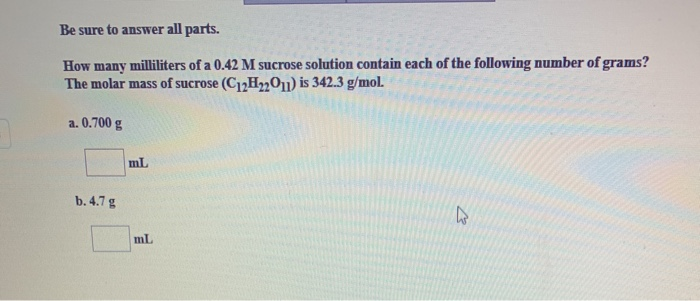Be sure to answer all parts. How many milliliters of a 0.42 M sucrose solution contain each of the following number of grams? The molar mass of sucrose (C12H2011) is 342.3 g/mol a. 0.700 g ml b.4.7 g

• ### 2 attempts left Check my work Be sure to answer all parts. milliliters of a 0.17 M sucrose solution contain each of...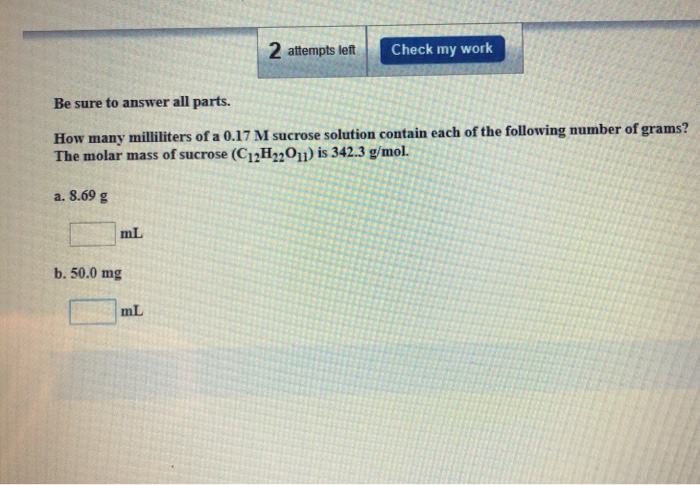2 attempts left Check my work Be sure to answer all parts. milliliters of a 0.17 M sucrose solution contain each of the following number of grams? How many The molar mass of sucrose (C12H22O11) is 342.3 g/mol. a. 8.69 g mL b. 50.0 mg mL

• ### Be sure to answer all parts. For an aqueous solution of sucrose (C12H22011), determine: (a) the...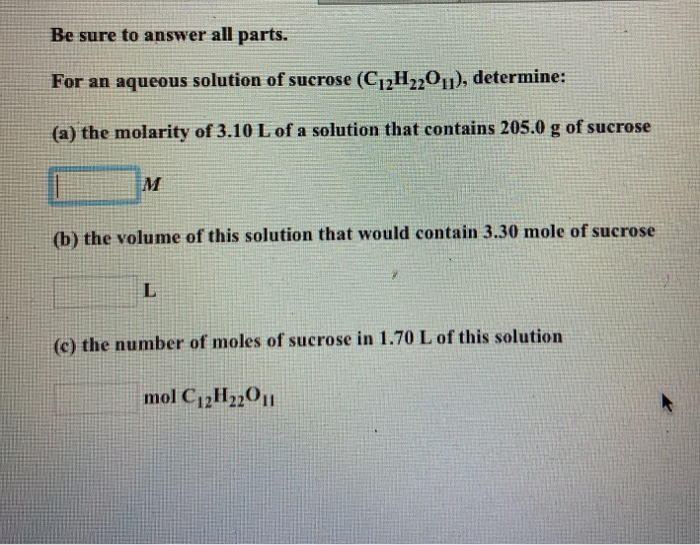Be sure to answer all parts. For an aqueous solution of sucrose (C12H22011), determine: (a) the molarity of 3.10 L of a solution that contains 205.0 g of sucrose M (b) the volume of this solution that would contain 3.30 mole of sucrose (c) the number of moles of sucrose in 1.70 L of this solution mol C12H220,1

• ### Be sure to answer all parts. How many moles are contained in each number of grams...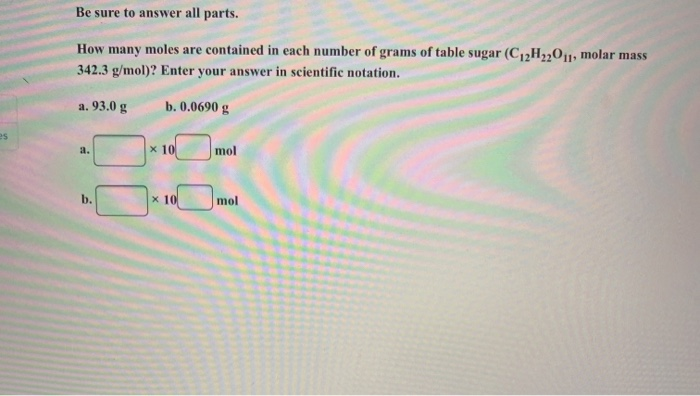Be sure to answer all parts. How many moles are contained in each number of grams of table sugar (C12H2,011, molar mass 342.3 g/mol)? Enter your answer in scientific notation. a. 93.0 g b. 0.0690 g mol

• ### Be sure to answer all parts. How many moles are contained in each number of grams...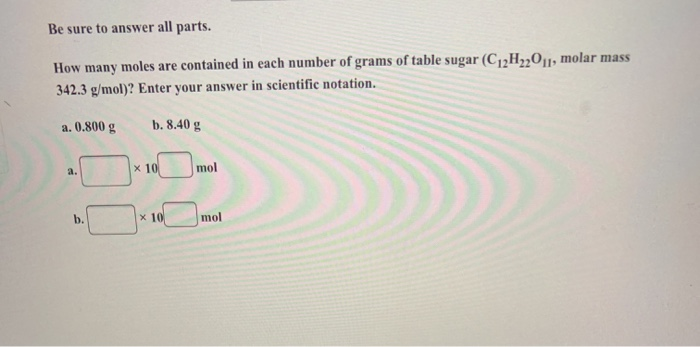Be sure to answer all parts. How many moles are contained in each number of grams of table sugar (C2H,201, molar mass 342.3 g/mol)? Enter your answer in scientific notation. a. 0.800 g b. 8.40 g mol

• ### Help 3 attempts left Check my work Be sure to answer all parts. Report problem points...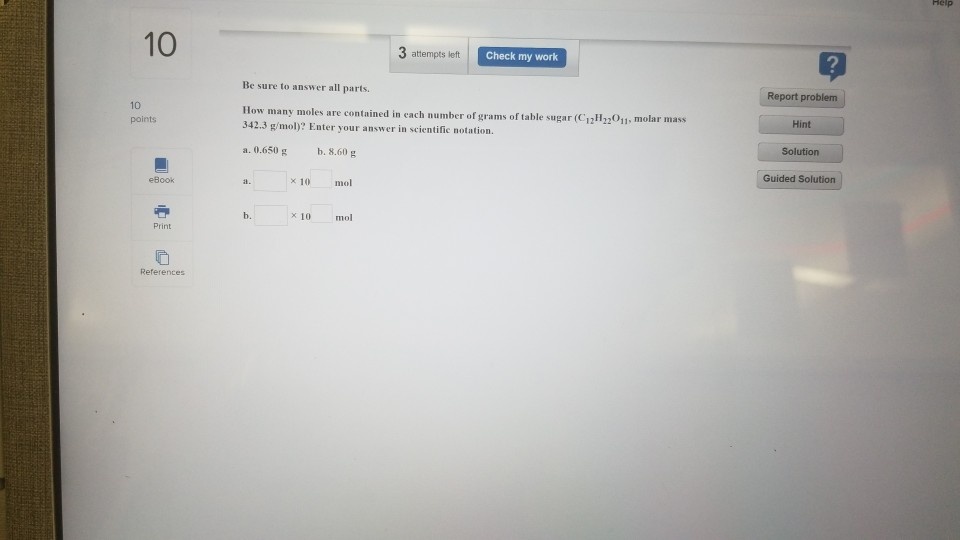Help 3 attempts left Check my work Be sure to answer all parts. Report problem points How many moles are contained in each number of grams of table sugar (C211,201 molar mass 342.3 g/mol)? Enter your answer in scientific notation. Hint a. 0.650 g b . 8.60 g Solution eBook a 10 mol Guided Solution b. 10 mol Print References

• ### Isopropyl alcohol is mixed with water to produce a 38.0% (v/v) alcohol solution. How many milliliters...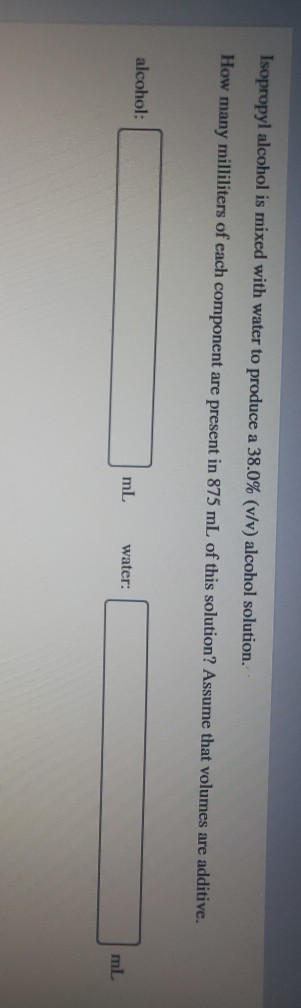Isopropyl alcohol is mixed with water to produce a 38.0% (v/v) alcohol solution. How many milliliters of each component are present in 875 mL of this solution? Assume that volumes are additive. alcohol: mL water: ml The mass of solute per 100 mL of solution is abbreviated as (m/v). Mass is not technically the same thing as weight, but the abbreviation (w/v) is also common. How many grams of sucrose are needed to make 695 mL of a 38.0% (w/v)...

• ### How many moles of KMnO4 are present in 125 mL of a 0.0850 M solution? 0.011...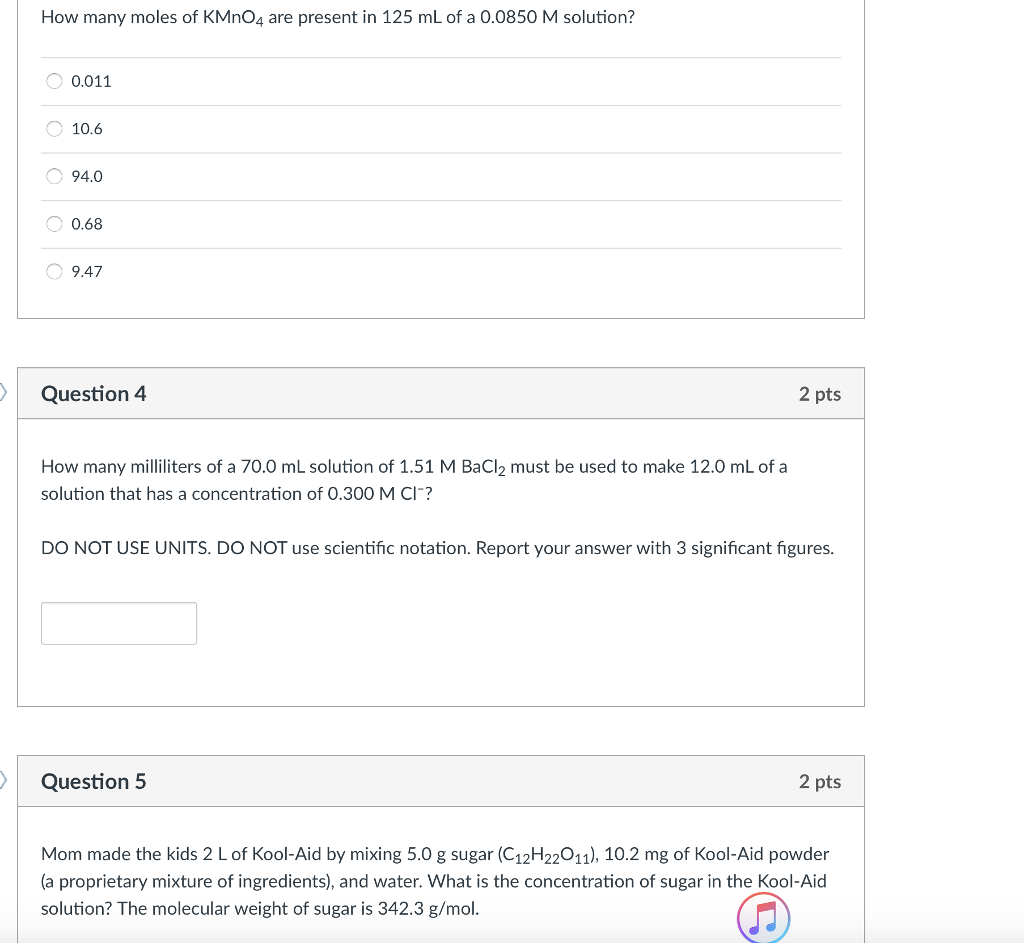How many moles of KMnO4 are present in 125 mL of a 0.0850 M solution? 0.011 10.6 94.0 0.68 9.47 Question 4 2 pts How many milliliters of a 70.0 mL solution of 1.51 M BaCl2 must be used to make 12.0 mL of a solution that has a concentration of 0.300 M CI? DO NOT USE UNITS. DO NOT use scientific notation. Report your answer with 3 significant figures. Question 5 2 pts Mom made the kids 2 L...

• ### 3a. How many grams of sucrose must be added to 430g of water to lower the vapor pressure 1.4mmHg at 25C? The vapor press...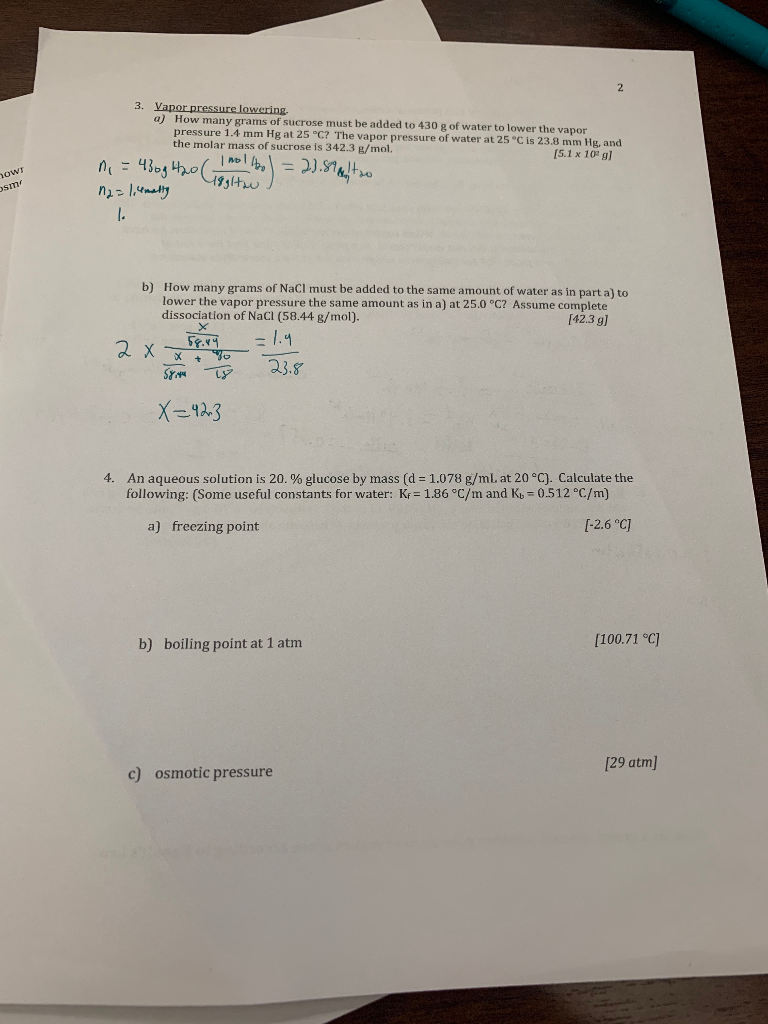3a. How many grams of sucrose must be added to 430g of water to lower the vapor pressure 1.4mmHg at 25C? The vapor pressure of water at 25C is 233.8 mmHg and molar mass is 342.3 g/mol 3. Vapor pressure lowering a) How many grams of sucrose must be added to 430 R of water to lower the vapor pressure 1.4 mm Hg at 25 °C? The vapor pressure of water at 25°C is 23.8 mm Hg and the molar...

• ### The nonvolatile, nonelectrolyte sucrose , C12H22O11 (342.3 g/mol), is soluble in water H2O. How many grams...

The nonvolatile, nonelectrolyte sucrose , C12H22O11 (342.3 g/mol), is soluble in water H2O. How many grams of sucrose are needed to generate an osmotic pressure of 3.56 atm when dissolved in 227 ml of a water solution at 298 K. grams sucrose